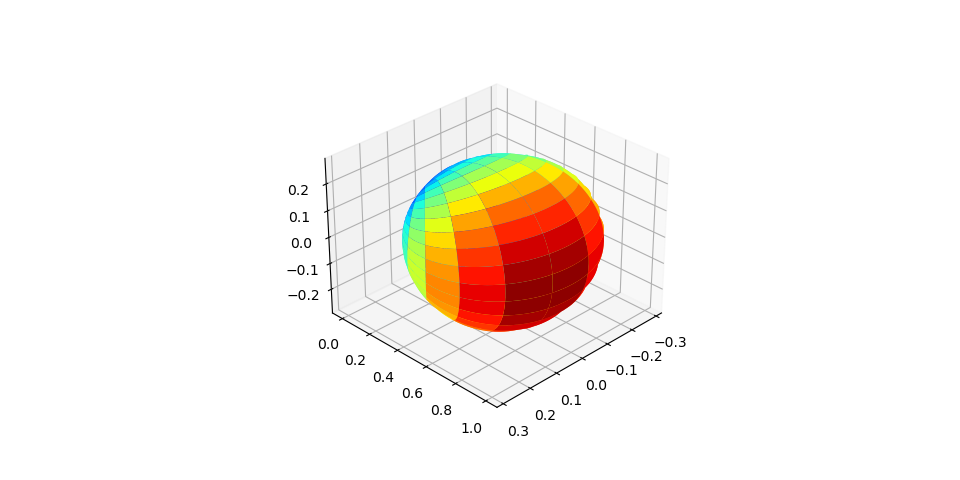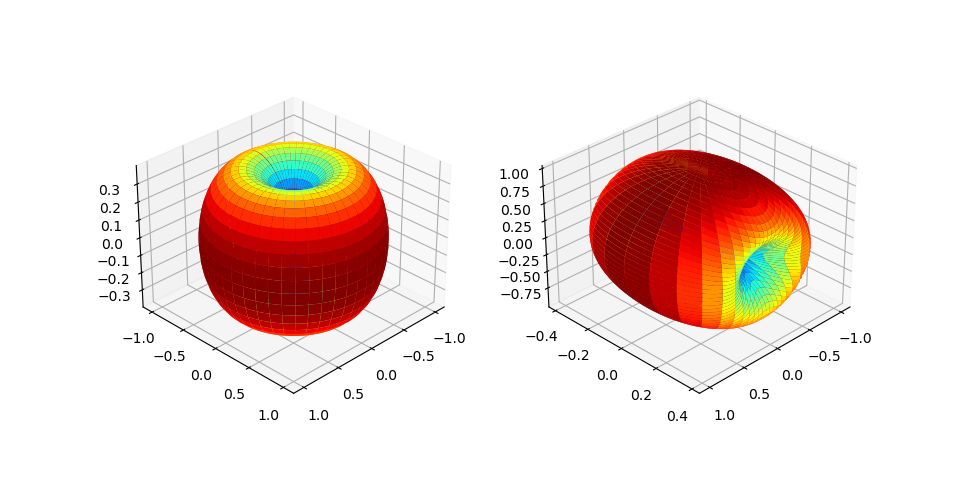# Antenna Array

This module provides classes and the functions that implements Antenna Array.

Antenna Array is a set of multiple connected antennas, called as antenna elements that work together as a single antenna. Current implementation support different types of antenna elements such as 3GPP 38.901, Hertzian Dipole and Linear Dipole, different types of array structures such as Uniform Linear Array (ULA), Uniform Planar Array (UPA) and Multi Panel Array (MPA) as specified in 3GPP TR 38.901. The class `AntennaArrays` implement the MPA.

class toolkit5G.ChannelModels.AntennaArrays(antennaType='OMNI', centerFrequency=3 * 10**9, arrayStructure=np.array([1, 1, 1, 1, 1], dtype=np.uint16), antennaSpacing=np.array([0.5, 0.5, 0.5, 0.5]), antParameters=None, phaseDiff=0, thetaGrid=np.arange(0, pi, pi / 180), phiGrid=np.arange(-pi, pi, pi / 180))[source]

A class for generating Antenna Arrays.

This class supports different types of antenna element such as “3GPP_38.901”, “HERTZIAN DIPOLE” and “LINEAR DIPOLE”, and different types of array structures such as Uniform Linear Array (ULA), Uniform Planar Array (UPA) and Multi Panel Array (MPA).

Current implementation support both single and dual polarized antenna. Ref: TR 38.901 for “3GPP_38.901” antenna specifications including MPA. Some important features of this class are displaying radiation pattern (both in 2D and 3D) using a method displayAntennaRadiationPattern(). Displaying array factor (both 3D,2D including surface or polar plot) using a method displayAntennaArrayFactor(). Rotating radiation pattern using rotateAntennaRadiationPattern() method by passing 3 angles [alpha, beta, gama] (also called as Euler Angles) per panel.

Parameters:
• antennaType (str) – Specifies the type of antenna element. Supported antennaType are “OMNI” or “3GPP_38.901” or “HERTZIAN_DIPOLE” or “LINEAR_DIPOLE”.

• centerFrequency (int/float) – Carrier frequency in Hz. Defaults to 3*10**9 Hz (i.e., 3 GHz). Must be always a positive int or a float.

• arrayStructure (NumPy.ndarray of int) –

Antenna Array Geometry, an Array of 5 integers in sequence. i.e., [`Mg`, `Ng`, `M`, `N`, `P`], where

• `Mg` : Number of Panel in Vertical Direction. Must be a positive int greater than 0.

• `Ng` : Number of Panel in Horizontal Direction. Must be a positive int greater than 0.

• `M` : Number of antenna elements (with same polarization) per column per panel. Must be a positive int greater than 0.

• `N` : Number of columns per panel (= number of ULA’s). Must be a positive int greater than 0.

• `P` : Antenna Polarization. Must be a positive int either 1 or 2. (P = 1 for single polarized antenna element and P = 2 for dual polarized antenna element)

Defaults to numpy.array([1,1,1,1,1]).

• antennaSpacing (NumPy.ndarray of float) –

Specifies spacing between panel and spacing between antenna elements, an Array of 4 ints or floats. i.e., [`dgv`, `dgh`, `dv`, `dh`], where

• `dgv` : Vertical spacing between any two panel. Must be a positive int or float greater than 0.

• `dgh` : Horizontal spacing between any two panel. Must be a positive int or float greater than 0.

• `dv` : Vertical spacing between any two antenna element. Must be a positive int or float greater than 0.

• `dh` : Horizontal spacing between any two antenna element. Must be a positive int or float greater than 0.

Defaults to numpy.array([0.5,0.5,0.5,0.5]).

• antParameters (NumPy.ndarray/int/float) –

Parameters specific to each antenna element of an array. Defaults to None

• For antenna element 3GPP_38_901, an array of 4 ints or floats correspond to

• `theta_3dB` - Half power beamwidth along elevation direction, defaults to 65 in degrees. Any positive int or float greater than 0 is valid.

• `SLAv` - Vertical Side Lobe Attenuation in dB, defaults to 30 dB. Any positive int or float greater than 0 is valid.

• `phi_3dB` - Half power beamwidth along azimuth direction, defaults to 65 in degrees. Any positive int or float greater than 0 is valid.

• `Amax` - Max Radiation Power in dB, defaults to 30 dB. Any positive int or float greater than 0 is valid.

Defaults to numpy.array([65/180*pi, 30, 65/180*pi, 30])

• For Hertzian or Linear Dipole, an int or float corresponds to `length` of antenna element Defaults to None. Any positive int or float greater than 0 is valid. Note that antenna element `length` is always a multiple of carrier wave length.

• phaseDiff (float) – Phase Difference between antenna elements of a Uniform Linear Array (ULA). Defaults to 0 in rad. Any int or float is valid.

• thetaGrid (NumPy.ndarray) – Range of Theta’s (zenith angles) in Radians. Defaults to numpy.arange(0, pi, pi/180).

• phiGrid (NumPy.ndarray) – Range of Phi’s (azimuth angles) in Radians. Defaults to numpy.arange(-pi, pi, pi/180).

Input:
• orientationperPanel (NumPy.ndarray of ints or floats) –

antenna array orientations are an array of 3 angles correponding to each panel. i.e., [alpha,beta,gama]

• alpha - bearing angle in rad.

• beta - down-tilt angle in rad.

• gama - slant angle in rad.

Defaults to np.array([[0, 0, 0]]) corresponds to all the panels having the same [alpha,beta,gama]. This numpy array can take a shape of either (1,3) or (numOfPanel,3), where numOfPanel = Mg*Ng.

• polarizationSlantAngle (int/float/NumPy.ndarray of ints or floats.) – Polarization slant angle in rad for each antenna element of a panel. Defaults to 0 corresponds to all the antenna elements of each panel are having the same polarization slant angle. When passed as a numpy array it can take a shape of either (1,) or (numOfPanel,)

• directionalGainMax (int) – Maximum directional gain of an antenna element. Must be a positive int and defaults to 8 dBi.

Raises:
• ValueError – If `antennaType` is not a str type / the passed `antennaType` is not from the supported antenna type list [“OMNI”, “3GPP_38.901”, “HERTZIAN_DIPOLE”, “LINEAR_DIPOLE”].

• ValueError – If `centerFrequency` is not a positive number / positive number and not an int/float type.

• ValueError – If `arrayStructure` is not a numpy array / A numpy array of not having 5 non zero integers corresponding to [Mg,Ng,M,N,P].

• ValueError – If `antennaSpacing` is not a numpy array / A numpy array of not having 4 non zero integers corresponding to [dgv,dgh,dv,dh].

• ValueError – If `orientationperPanel` is neither a scalar nor numpy array. If scalar and not an instance of either int or float type. If numpy array with a shape other than (1,3) or (numOfPanel,3), where numOfPanel = Mg*Ng

• ValueError – If `polarizationSlantAngle` is neither a scalar nor numpy array. If scalar and not an instance of either int or float type. If numpy array with a shape other than (numOfPanel,)

Example:

3GPP_38.901 Antenna Array

In the following code snippet only one array of angles [alpha,beta,gama] = [0,0,0] passed. i.e., all the panels are assumed to have the same orienatation. The num of orientation angles can be either one or num of panel and should not exceed the num of panel Mg*Ng = 1. i.e., `orientationperPanel` is a numpy array with dimension (1,3) or (Mg*Ng,3).

Also here only one `polarizationSlantAngle` (psa) = 0 passed. i.e., all the antenna elements per panel are assumed to have the same psa 0 radians. The num of psa can be either one or num of panel and should not exceed the num of panel Mg*Ng = 1. i.e., psa is either a scalar (int or float) or a numpy array of shape (Mg*Ng,).

```>>> antArrayObj = AntennaArrays(antennaType     = "3GPP_38.901",
...                             centerFrequency = 3*10**9,
...                             arrayStructure  = np.array([1, 1, 1, 1, 1]),
...                             antennaSpacing  = np.array([0.5, 0.5, 0.5, 0.5]))
>>> antArrayObj(orientationperPanel    = np.array([[0, 0, 0]]),
polarizationSlantAngle = 0)
```

The following figure displays the radiation pattern of a 3GPP_38.901 antenna element. User can display such plot by calling the method `displayAntennaRadiationPattern()`Hertzina Dipole Antenna Array

In the following code snippet the num of orientation angles equals the number of panel Mg*Ng = 2. The num of orientation angles can be either one or num of panel and should not exceed the num of panel. Here the num of psa is also 2. The num of psa can be either one or num of panel and should not exceed the num of panel Mg*Ng = 2.

```>>> antArrayObj = AntennaArrays(antennaType     = "HERTZIAN_DIPOLE",
...                             centerFrequency = 3*10**9,
...                             arrayStructure  = np.array([1, 2, 1, 1, 1]),
...                             antennaSpacing  = np.array([0.5, 0.5, 0.5, 0.5]))
>>> antArrayObj(orientationperPanel    = np.array([[0, 0, 0], [90*np.pi/180,90/180*np.pi,90*np.pi/180]]),
polarizationSlantAngle = np.array([0,90*np.pi/180]))
```

The radiation pattern for this example looks as follows:OMNI Antenna Array

In this example, We instantiate `AntennaArrays` class with antenna type as “OMNI” with antParameters which is length of antenna element = c/fc. i.e., 3*10**8/3*10**9 for “OMNI”.

```>>> antArrayObj = AntennaArrays(antennaType     = "OMNI",
centerFrequency = 3*10**9,
arrayStructure  = np.array([1, 1, 1, 1, 1]),
antennaSpacing  = np.array([0.5, 0.5, 0.5, 0.5]),
antParameters   = 3*10**8/3*10**9)
>>> antArrayObj(orientationperPanel    = np.array([[0, 0, 0]]),
polarizationSlantAngle = 0)
```

## Antenna Elements

This module provides classes and the functions that implements antenna elements of an array. The class `Antenna_3GPP_38901` implements the 3GPP_38901 antenaa element specified in TR 38.901. Classes `Antenna_HertzianDipole` and `Antenna_LinearDipole` implement Hertzian, Linear Dipole antenna element respectively.

### 3GPP_38_901 Antenna Element

class toolkit5G.ChannelModels.antennaArrays.Antenna_3GPP_38901(theta_3dB=65 / 180 * pi, SLAv=30, phi_3dB=65 / 180 * pi, Amax=30, thetaGrid=np.arange(0, pi, pi / 180), phiGrid=np.arange(0, pi, pi / 180), directionalGainMax=8)[source]

A class which implements antenna element 3GPP_38901 specified in TR 38.901.

Parameters:
• theta_3dB (int/float) – Half power beamwidth along elevation angle. Must be either an int/float. Defaults to 65/180*pi, i.e., 65 degree in radians.

• SLAv (int/float) – Side-lobe attenuation in vertical direction. Usually specified in dBi and defaults to 30.

• phi_3dB (int/float) – Half power beamwidth along azimuth angle. Must be either an int/float. Defaults to 65/180*pi, i.e., 65 degree in radians.

• Amax (int/float) – Maximum antenna gain in dBi. Defaults to 30.

• thetaGrid (NumPy.ndarray of float) – Array of angles in elevation/zenith direction. Defaults to np.arange(0, pi, pi/180).

• phiGrid (NumPy.ndarray of float) – Array of angles in azimuth direction. Defaults to np.arange(0, pi, pi/180).

• directionalGainMax (int) – Maximum directional gain of an antenna element. Ge_max from Table 7.3-1 of 3GPP TR 38.901. Must be a positive int and defaults to 8 dBi.

### Hertzian Dipole Antenna Element

class toolkit5G.ChannelModels.antennaArrays.Antenna_HertzianDipole(carrierFrequency=3 * 10**9, length=None, thetaGrid=np.arange(0, np.pi, np.pi / 180, dtype=np.float32), phiGrid=np.arange(-np.pi, np.pi, np.pi / 180, dtype=np.float32))[source]

A class which implements Hertzian Dipole antenna element.

Parameters:
• carrierFrequency (int/float) – Carrier Frequency at which the dipole antenna function. Must be either an int/float and has to be positive. Defaults to 3*10**9. i.e., 3 GHz.

• length (None/int/float.) – length of hertzian dipole antenna. Defaults to None. In the default case length is initialized to lamda/10, where lamda is the carrier wave length. Must be always positive if either int/float.

• thetaGrid (NumPy.ndarray of float) – Array of angles in elevation/zenith direction. Defaults to np.arange(0, pi, pi/180).

• phiGrid (NumPy.ndarray of float) – Array of angles in azimuth direction. Defaults to np.arange(-pi, pi, pi/180).

### Linear Dipole Antenna Element

class toolkit5G.ChannelModels.antennaArrays.Antenna_LinearDipole(carrierFrequency=3 * 10**9, length=None, thetaGrid=np.arange(0, np.pi, np.pi / 180, dtype=np.float32), phiGrid=np.arange(-np.pi, np.pi, np.pi / 180, dtype=np.float32))[source]

A class which implements Linear Dipole antenna element.

Parameters:
• carrierFrequency (int/float) – Carrier Frequency at which the dipole antenna function. Must be either an int/float and has to be positive. Defaults to 3*10**9. i.e., 3 GHz.

• length (None/int/float.) – length of hertzian dipole antenna. Defaults to None. In the default case length is initialized to lamda/4, where lamda is the carrier wave length. Must be always positive if either int/float.

• thetaGrid (NumPy.ndarray of float) – Array of angles in elevation/zenith direction. Defaults to numpy.arange(0, pi, pi/180).

• phiGrid (NumPy.ndarray of float) – Array of angles in azimuth direction. Defaults to numpy.arange(-pi, pi, pi/180).

Note

All the classes have the following attributes `fieldTheta`, `fieldPhi` from which the user can access the antenna field vectors in zenith, azimuth directions respectively.